#2018 iT 邦幫忙鐵人賽DAY 29
0
Software Development

Protocol好長啊。大挑戰Ｒ

# 協定 Protocol

## 協定語法

``````protocol SomeProtocol {
protocol 定義的內容
}
``````

``````struct SomeStructure: SomeProtocol, AnotherProtocol {
// struct 定義的內容
}
``````

``````class SomeClass: SomeSuperclass, SomeProtocol, AnotherProtocol {
// class 定義的內容
}
``````

## 屬性要求

``````protocol SomeProtocol {
var readOnly: Int { get } // 唯讀變數
var readAndWritable: Int { get set } // 可讀寫變數
}
``````

``````protocol AnotherProtocol {
static var someTypeProperty: Int { get set }
}
``````

``````protocol FullyNamed {
var fullName: String { get }
}
``````

``````struct Person: FullyNamed {
var fullName: String
}

let jeremy = Person(fullName: "Jeremy Xue")
``````

``````class ChineseName:FullyNamed {
var lastName:String?
var name: String
init(name:String,lastName:String? = nil) {
self.name = name
self.lastName = lastName
}
var fullName: String{
return name + " " + (lastName != nil ? lastName!:"")
}
}
``````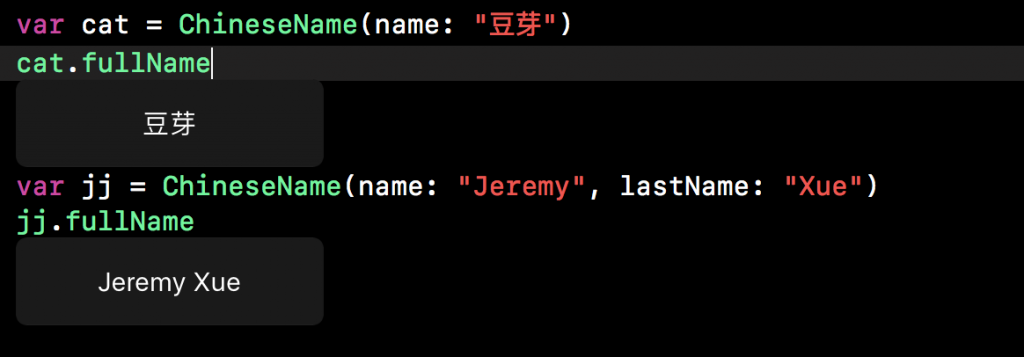## 方法要求

``````protocol SomeProtocol {
static func someTypeMethod()
}
``````

``````protocol Fibonacci {
func calculate() -> Int
}
``````

``````class FibonacciNum:Fibonacci{
var a = 0
var b = 1
var total = 0

func calculate() -> Int {
total = a + b
a = b
b = total
print(total)
return total
}
}
``````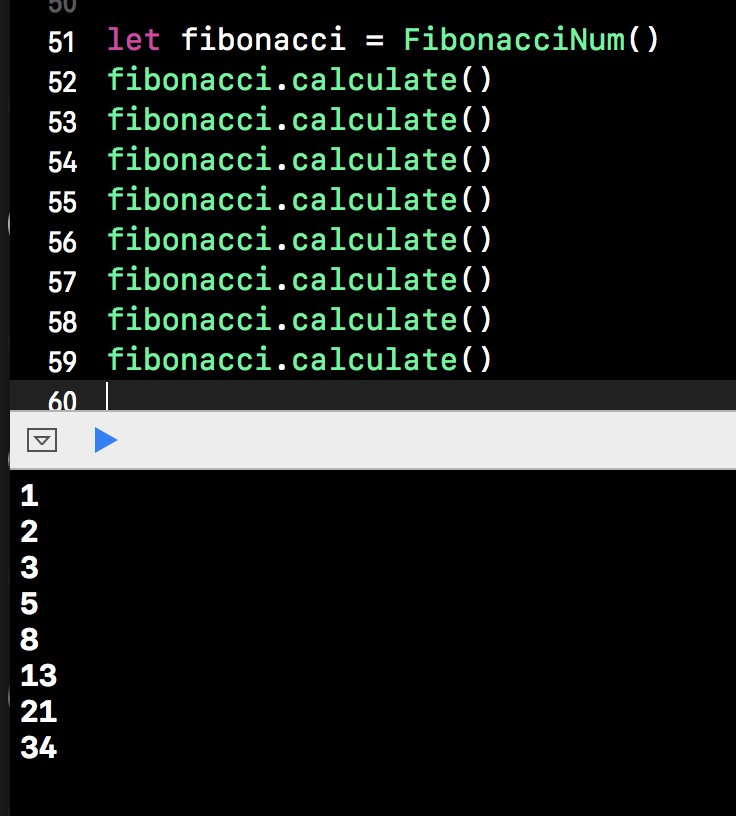# 異變方法要求

``````protocol Change {
mutating func change()
}
``````

``````enum DirectionChange:Change {
case up, down, left, right
mutating func change() {
switch self {
case .up:
self = .down
print("方向顛倒，現在是下")
case .down:
self = .up
print("方向顛倒，現在是上")
case .left:
self = .right
print("方向顛倒，現在是右")
case .right:
self = .left
print("方向顛倒，現在是左")
}
}
}
``````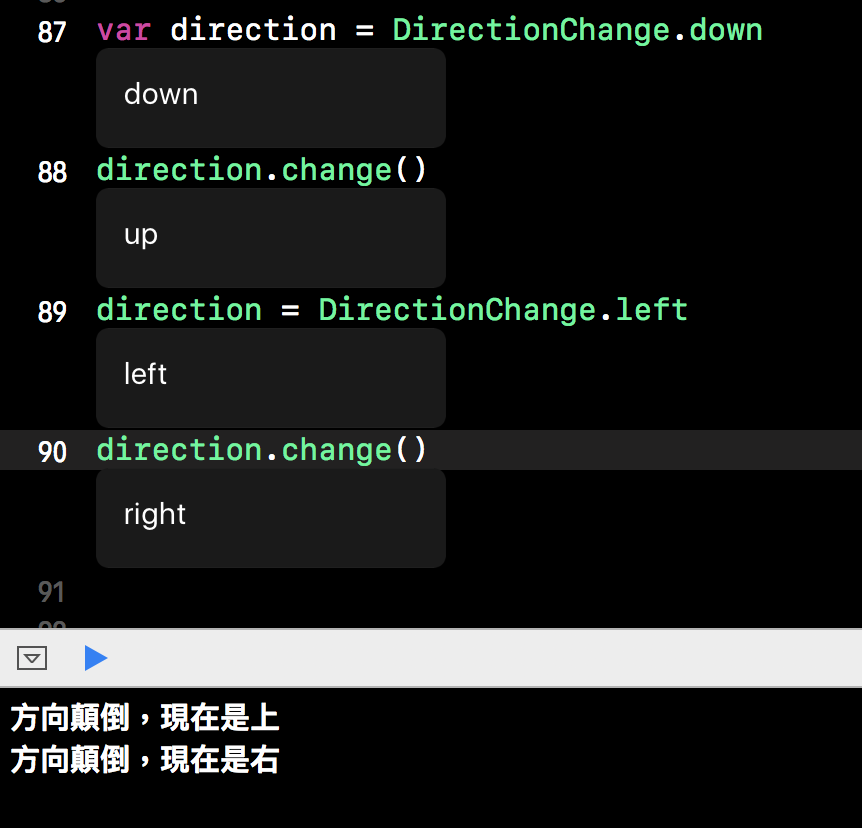## 初始化器要求

``````protocol SomeProtocol {
init(someParameter: Int)
}
``````

### 協議初始化器要求的類實現

``````class SomeClass: SomeProtocol {
required init(someParameter: Int) {
// 初始化內容
}
}
``````

``````protocol SomeProtocol {
init()
}

class SomeSuperClass {
init() {

}
}

class SomeSubClass:SomeSuperClass, SomeProtocol {
required override init() {

}
}
``````

## 將協議作為類型

• 在函數、方法或者初始化器裡作為形式參數類型或者返回類型；
• 作為常數、變數或者屬性的類型；
• 作為數組、字典或者其他存儲器的元素的類型。

``````protocol SomeProtocol {
func msg() -> String
}

class SomeClass:SomeProtocol {
func msg() -> String {
return "協議作為類型"
}
}

class AnotherClass {
let msg:SomeProtocol
init(msg:SomeProtocol) {
self.msg = msg
}
}
``````

``````let SomeInstance = SomeClass()
let AnotherInstance = AnotherClass(msg: SomeInstance)
``````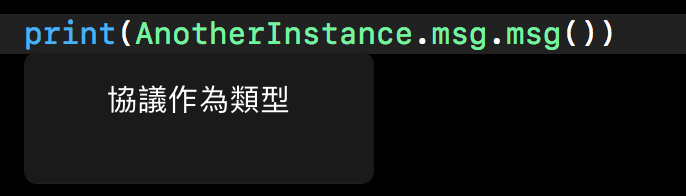## 委託

``````protocol Calculate {
func result()
}

protocol NewValue {
func newValue()
}

var a = 0
var b = 1
var c = 0

class Fibonacci:Calculate {
var delegate:NewValue?

func result() {
c = a + b
print(c)
delegate?.newValue()
}

}

class FibonacciValue:NewValue {
func newValue() {
a = b
b = c
}
}

let fib = Fibonacci()
let fibValue = FibonacciValue()
fib.delegate = fibValue

fib.result()
``````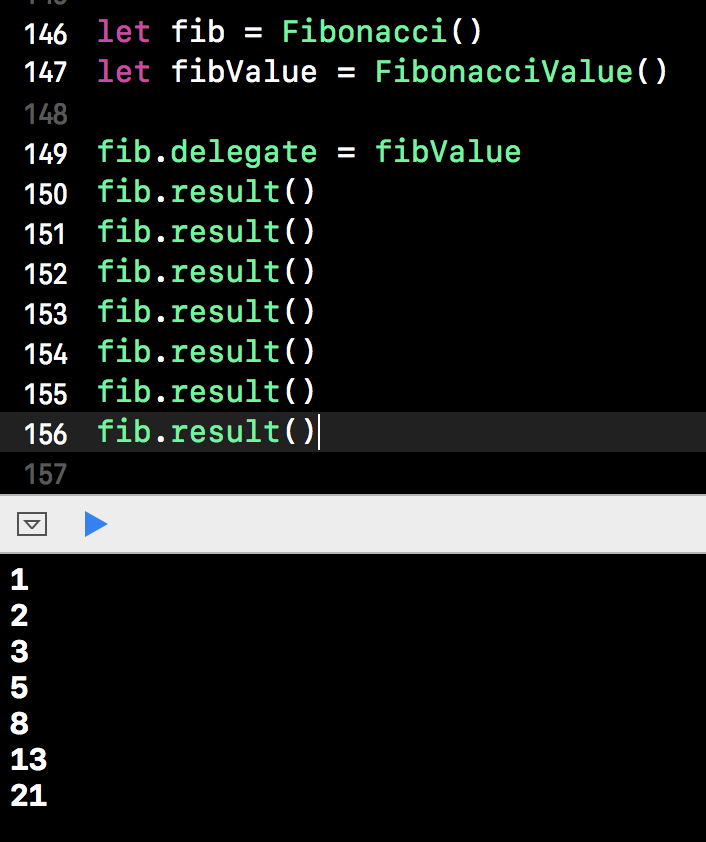## 使用擴展聲明採納協議

``````protocol Value {
func value()
}

extension Fibonacci:Value {
func value() {
print("現在 a 的值為：\(a)")
print("現在 b 的值為：\(b)")
}
}
``````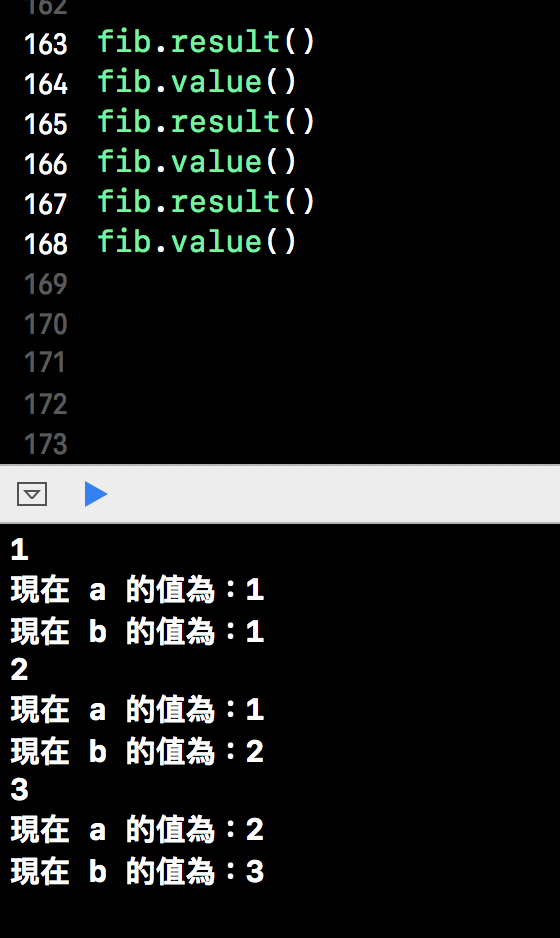## 使用擴展宣告採納協議

``````protocol SomeProtocol {
var name: String {get set}
}
// 定義一個 class 滿足了 SomeProtocol 的要求，但尚未採用它
class SomeClass {
var name = "Jeremy"
}
// 透過擴展宣告採用協議
extension SomeClass:SomeProtocol {}
``````

Swift 菜鳥的30天30